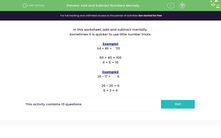# Add and Subtract Numbers Mentally

In this worksheet, students practise adding and subtracting numbers mentally.Key stage:  KS 2

Curriculum topic:   Number: Addition, Subtraction, Multiplication and Division

Curriculum subtopic:   Perform Mental Calculations

Popular topics:   Subtraction worksheets, Addition worksheets

Difficulty level:#### Worksheet Overview

In this worksheet, add and subtract mentally.

Sometimes it is quicker to use little number tricks.

Example1

64 + 46 =
 110

60 + 40 = 100

4 + 6 = 10

Example2

26 − 17 =
 9

26 - 20 = 6

6 + 3 = 9

### What is EdPlace?

We're your National Curriculum aligned online education content provider helping each child succeed in English, maths and science from year 1 to GCSE. With an EdPlace account you’ll be able to track and measure progress, helping each child achieve their best. We build confidence and attainment by personalising each child’s learning at a level that suits them.

Get started